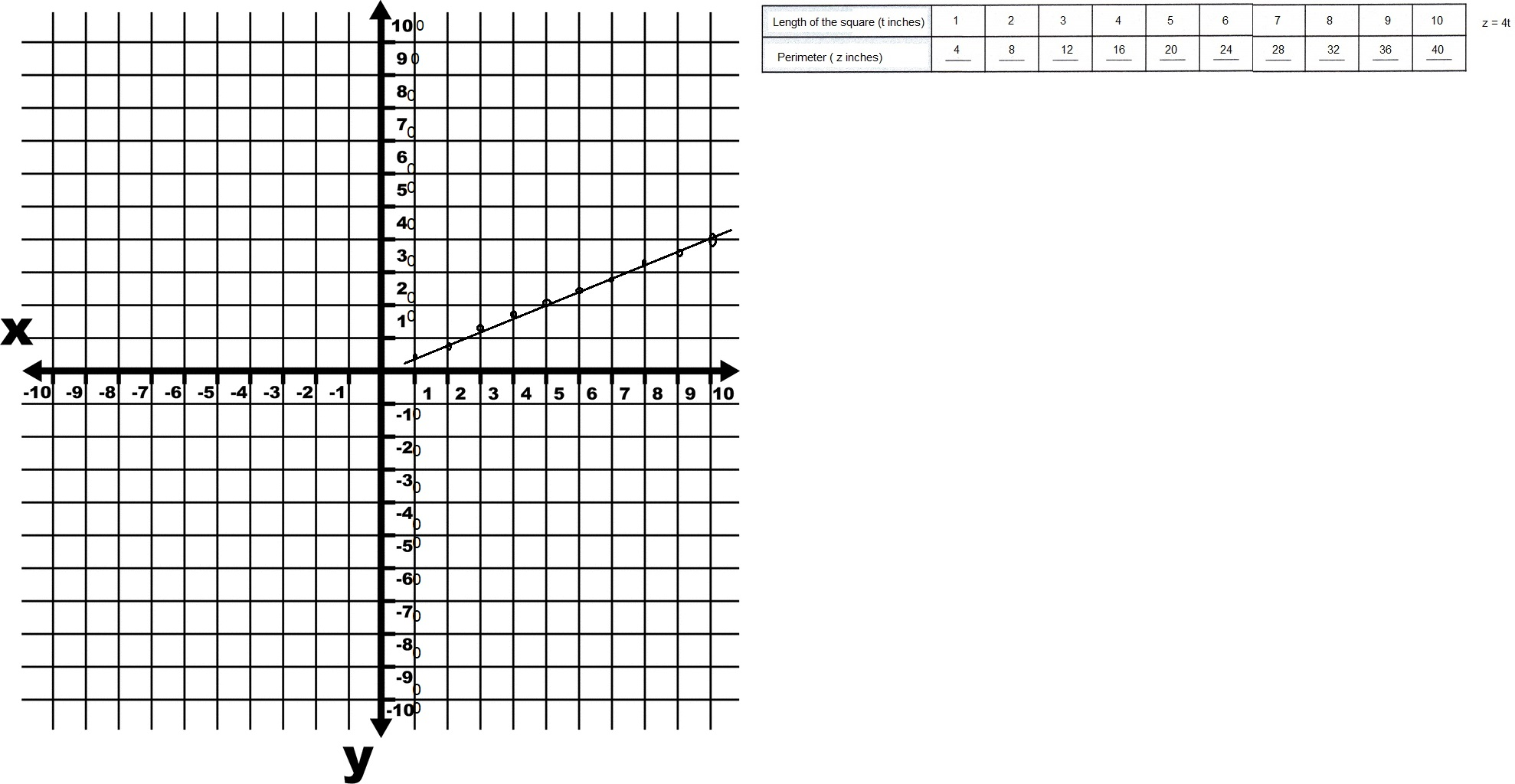# Math in Focus Grade 6 Chapter 8 Lesson 8.2 Answer Key Writing Linear Equations

Practice the problems of Math in Focus Grade 6 Workbook Answer Key Chapter 8 Lesson 8.2 Writing Linear Equations to score better marks in the exam.

## Math in Focus Grade 6 Course 1 B Chapter 8 Lesson 8.2 Answer Key Writing Linear Equations

### Math in Focus Grade 6 Chapter 8 Lesson 8.2 Guided Practice Answer Key

Complete.

Question 1.
Isaiah has h baseball cards. Miguel has 7 more baseball cards than Isaiah.
a) Write an expression for the number of baseball cards that Miguel has in terms of h.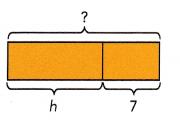Miguel has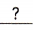baseball cards.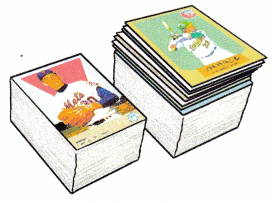b) If Miguel has k baseball cards, express k in terms of h.
k =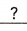+c) State the independent and dependent variables.
Independent variable:, dependent variable:a) Miguel has h + 7 baseball cards,
b) k = h + 7,
c) Independent variable: h, dependent variable: k,

Explanation:
Given Isaiah has h baseball cards. Miguel has 7 more baseball cards than Isaiah,
a) Wrote an expression for the number of baseball cards that Miguel has in terms of h as Miguel has h + 7 baseball cards.
b) If Miguel has k baseball cards expressing k in terms of h as k = h + 7.
c) The independent and dependent variables are independent variable is h and dependent variable are k which depends on value of h.

Write an equation for each of the following. Then state the independent and dependent variables for each equation.

Question 2.
Hannah took p minutes to jog around a park. Sofia took 12 minutes longer to jog around the park. If Sofia took t minutes to jog around the park, express t in terms of p.
t = p + 12,
Independent  variable : p, Dependent variable: t,

Explanation:
Given Hannah took p minutes to jog around a park. Sofia took 12 minutes longer to jog around the park. If Sofia took t minutes to jog around the park,To jog around the park it is p minutes, and Sofia took 12 more minutes if Sofia took t minutes expressing t in terms of p it is t = p + 12. Here p is independent variable and t is dependent variable on the value of p.

Question 3.
A bouquet of roses costs $30. A bouquet of tulips costs m dollars less. If the cost of one bouquet of tulips is n dollars, express n in terms of m. Answer: n =$30 – m,
Independent variable : m, Dependent variable: n,

Explanation:
Given a bouquet of roses costs $30. A bouquet of tulips costs m dollars less. If the cost of one bouquet of tulips is n dollars, expressing n in terms of m as n =$30 – m, Here m is independent variable and n is dependent variable on value of m.

Question 4.
Nathan has 7 boxes of marbles. Each box contains b marbles. If he has c marbles altogether, express c in terms of b.
c = 7b,
Independent variable : b, Dependent variable: c,

Explanation:
Given Nathan has 7 boxes of marbles. Each box contains b marbles. If he has c marbles altogether, expressing c in terms of b as c = 7b as Nathan has 7 boxes of b marbles each here b is independent variable and c is dependent variable on the value of b.

Question 5.
A motel charges Mr. Kim x dollars for his stay. Mr. Kim stayed at the motel for 12 nights. If the rate per night for a room is y dollars, express y in terms of x.
x = 12y,
Independent variable : b, Dependent variable: c,

Explanation:
Given a motel charges Mr. Kim x dollars for his stay. Mr. Kim stayed at the motel for 12 nights. If the rate per night for a room is y dollars expressing y in terms of x as it is x = 12y where y is independent variable and x is dependent variable on the value of y.

Copy and complete the table. Then use the table to answer the questions.

Question 6.
The width of a rectangular tank is 2 meters less than its length.
a) If the length is p meters and the width is q meters, write an equation relating p and q.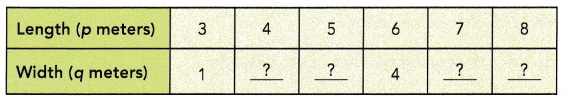Equation: q = p – 2,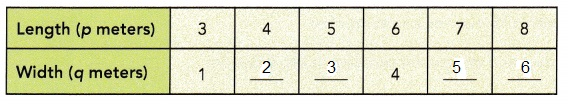Explanation:
Given The width of a rectangular tank is 2 meters less than its length.
a) If the length is p meters and the width is q meters so an equation relating p and q is q = p – 2,
If p =3 then q = 3 – 2 = 1,
If p = 4 then q = 4 – 2 = 2,
If p = 5 then q = 5 – 2 = 3,
If p = 7 then q = 7 – 2 = 5,
If p = 8 then q = 8 – 2 = 6,
Completed the table as shown above.

b) Use the data from a) to plot the points on a coordinate plane. Connect the points with a line.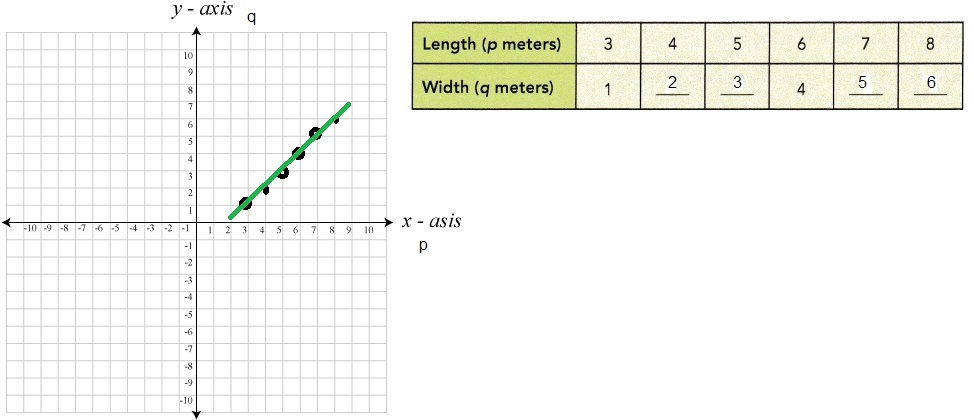Explanation:
Used the data from a) to plot the points on a coordinate plane as length p meters on x – axis and width q meters on y axis. Connected the points with a line as shown above.

c) The point (5.5, 3.5) is on the line you drew in b). Does this point make sense in the situation?
Yes,

Explanation:
The point(5.5,3.5) is on the line which i drew yes this point makes sense as p = 5.5 we have q = p – 2 = 5.5 – 2 = 3.5 which is true.

Copy and complete each table. Then express the relationship between the two variables as an equation.

Question 7.
Paul and Lee went to the library to borrow some books. Paul borrowed 6 more books than Lee.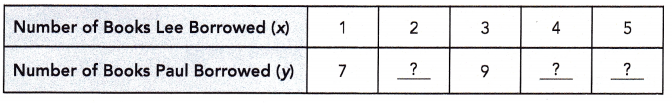Equation: y = x + 6,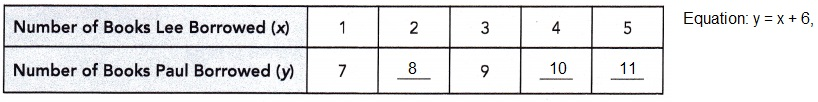Explanation:
Given Paul and Lee went to the library to borrow some books. Paul borrowed 6 more books than Lee. If x is number of books Lee borrowed and y be books Paul borrowed so the equation is y = x + 6, so
if x = 1 then y = 1 + 6 = 7 books,
if x = 2 then y = 2 + 6 = 8 books,
if x = 3 then y = 3 + 6 = 9 books,
if x = 4 then y = 4 + 6 = 10 books and
if x = 5 then y = 5 + 6 = 11 books shown in the above table.

Question 8.
At a crafts store, Zoey bought some boxes of red beads and some boxes of blue beads. The number of boxes of red beads was 4 times the number of boxes of blue beads.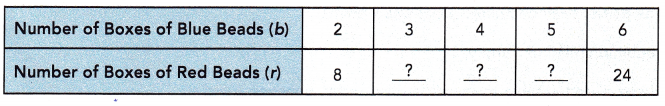Equation: r = 4b,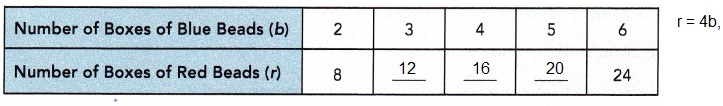Explanation:
Given at a crafts store Zoey bought some boxes of red beads and some boxes of blue beads. The number of boxes of red beads was 4 times the number of boxes of blue beads. Let read beads be r and blue beads be b so the equation is r = 4b,
if b = 2 then r = 4 X 2 = 8 beads,
if b = 3 then r = 4 X 3 = 12 beads,
if b = 4 then r = 4 X 4 = 16 beads,
if b = 5 then r = 4 X 5 = 20 beads shown above in the table.

Use the data in the table to plot points on a coordinate plane. Connect the points to form a line. Then write an equation to show the relationship between the variables.

Question 9.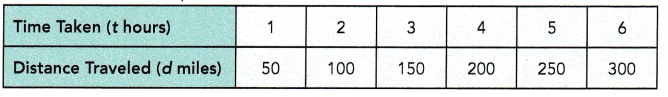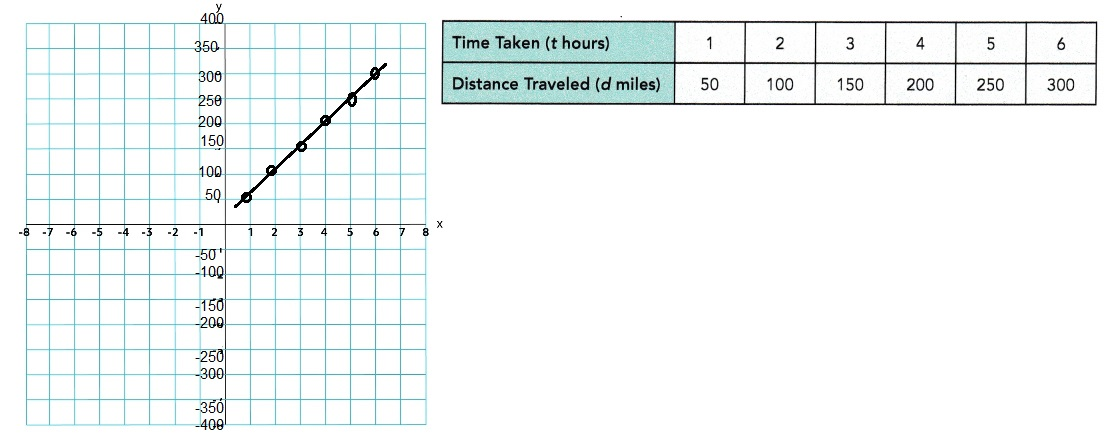Equation:
d = 50t,

Explanation:
Used the data in the table to plot points on a coordinate plane. Connected the points to form a line as shown above  here d is the distance traveled in miles on y – axis and t is the time taken in t hours on x – axis. As distance traveled is increasing by 50 each hour an equation to show the relationship between the variables is d = 50t.

### Math in Focus Course 1B Practice 8.2 Answer Key

Solve.

Question 1.
Joshua is w years old. His brother is 3 years older than he is.
a) If his brother is x years old, express x in terms of w.
x = w + 3,

Explanation:
Given Joshua is w years old. His brother is 3 years older than he is means brother is w + 3, So if his brother is x years old expressing x in terms of w as x = w + 3.

b) State the independent and dependent variables in the equation.
Independent variable : w,
Dependent variable : x,

Explanation:
Here Joshua is w years old his brother is 3 years older than he is and  if his brother is x years old then the independent variable is w and dependent variable is x on the value of w.

Question 2.
Rita has b markers. Sandy has 11 fewer markers than she has.

a) If Sandy has h markers, express h in terms of b.
h = b – 11,

Explanation:
As Rita has b markers. Sandy has 11 fewer markers than she has means Sandy has b – 11 markers, If Sandy has h markers expressing h in terms of b as h = b – 11.

b) State the independent and dependent variables in the equation.
Independent variable : b,
Dependent variable : h,

Explanation:
As Rita has b markers. Sandy has 11 fewer markers than she has means Independent variable is b and dependent variable is h on the value of b.

Question 3.
A small box of cereal weighs k grams. A jumbo box of cereal weighs 5 times as much.

a) If the weight of the jumbo box of cereal is m grams, express m in terms of k.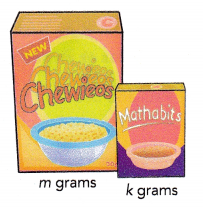m = 5k,

Explanation:
Given a small box of cereal weighs k grams. A jumbo box of cereal weighs 5 times as much means jumbo box is 5k, If the weight of the jumbo box of cereal is m grams, expressing m in terms of k as m = 5k.

b) State the independent and dependent variables in the equation.
Independent variable : k,
Dependent variable : m,

Explanation:
As a small box of cereal weighs k grams and a jumbo box of cereal weighs 5 times as much here the independent variable is k and dependent variable is m on the value of k.

Question 4.
The area of Hank’s farm is n acres. The area of Hank’s farm is 8 times as large as the area of Stan’s farm.

a) If s represents the area of Stan’s farm, express s in terms of n.
n = 8s,

Explanation:
Given the area of Hank’s farm is n acres. The area of Hank’s farm is 8 times as large as the area of Stan’s farm means Hank’s farm is 8 X Stan’s farm if s represents the area of Stan’s farm expressing s in terms of n is n = 8s.

b) State the independent and dependent variables in the equation.
Independent variable : s,
Dependent variable : n,

Explanation:
Given the area of Hank’s farm is n acres. The area of Hank’s farm is 8 times as large as the area of Stan’s farm means Hank’s farm is 8 X Stan’s farm if s represents the area of Stan’s farm expressing s in terms of n here Independent variable is s and dependent variable is n which depends on s.

Question 5.
Ethan scored x points in a game. His younger sister scored 8 points when she played the same game. Their combined score was y points.

a) Write an equation relating x and y.
y = x + 8,

Explanation:
Given Ethan scored x points in a game. His younger sister scored 8 points when she played the same game. Their combined score was y points. So an equation relating x and y is y = x + 8.

b) Copy and complete the table to show the relationship between x and y.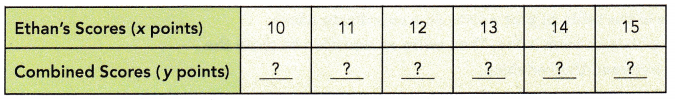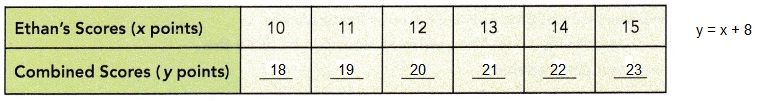Explanation:
Completed the table to show the relationship between x and y as y = x + 8,
if x = 10 then y = 10 + 8 = 18,
if x = 11 then y = 11 + 8 = 19,
if x = 12 then y = 12 + 8 = 20,
if x = 13 then y = 13 + 8 = 21,
if x = 14 then y = 14 + 8 = 22 and
if x = 15 then y = 15 + 8 = 23 as shown above.

Question 6.
There are x sparrows in a tree. There are 50 sparrows on the ground beneath the tree. Let y represent the total number of sparrows in the tree and on the ground.
a) Express y in terms of x.
y = x + 50,

Explanation:
Given there are x sparrows in a tree. There are 50 sparrows on the ground beneath the tree. Let y represent the total number of sparrows in the tree and on the ground.So expressing y in terms of x is y = x + 50.

b) Make a table to show the relationship between y and x. Use values of x = 10, 20, 30, 40, and 50 in your table.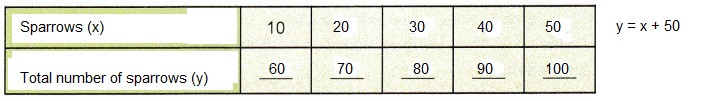Explanation:
Asked to make a table to show the relationship between y and x. Used values of x = 10, 20, 30, 40, and 50 in my table and completed as shown above in the equation y = x + 50,
When x = 10 then y = 10 + 50 = 60,
when x = 20 then y = 20 + 50 = 70,
when x = 30 then y = 30 + 50 = 80,
when x = 40 then y = 40 + 50 = 90,
when x = 50 then y = 50 + 50 = 100.

c) Graph the relationship between y and x in a coordinate plane.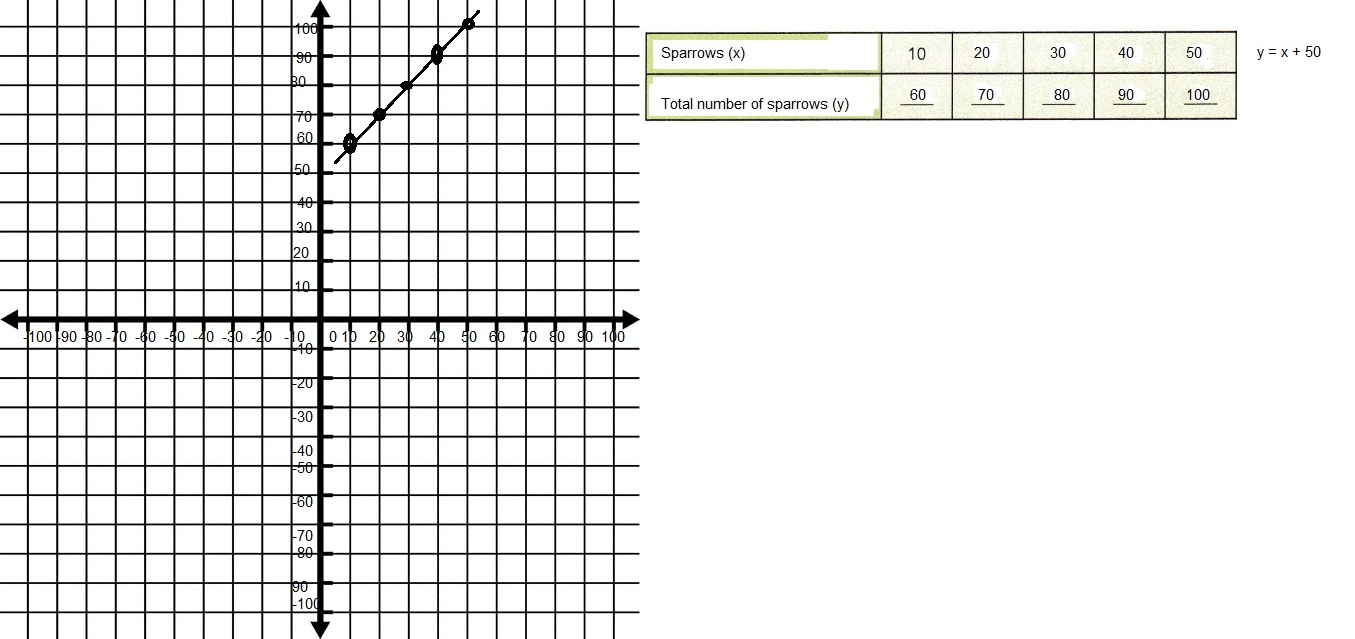Explanation:
Graphed the relationship between y and x in a coordinate plane as shown above as sparrows x on x -axis and total number of sparrows y on y – axis.

Question 7.
A rectangle has a perimeter of P centimeters. Its width is b centimeters. Its length is double its width.
a) Express P in terms of b.
P = 6b,

Explanation:
Given a rectangle has a perimeter of P centimeters. Its width is b centimeters. Its length is double its width.
So expressing P in terms of b as P = 2(2b + b). = 2(3b) = 6b.

b) Copy and complete the table to show the relationship between P and b.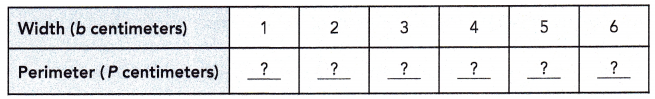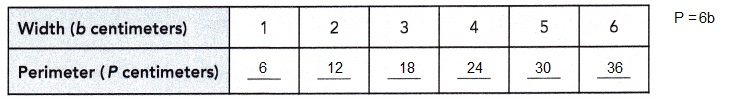Explanation:
Given a rectangle has a perimeter of P centimeters. Its width is b centimeters. Its length is double its width.
So expressed P in terms of b as P = 6b completed the table to show the relationship between P and b above as
if b= 1 then P = 6 X 1 = 6,
if b = 2 then P = 6 X 2 = 12,
if b = 3 then P = 6 X 3 = 18,
if b= 4 then P = 6 X 4 = 24,
if b = 5 then P = 6 X 5 = 30 and
if b = 6 then P = 6 X 6 = 36.

Question 8.
Every month, Amaan spends 60% of what he earns and saves the rest. Amaan earns n dollars and saves r dollars each month.
a) Express r in terms of n.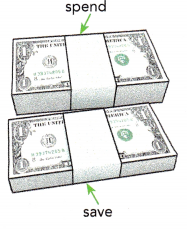r = n – 0.6n,

Explanation:
Given every month, Amaan spends 60% of what he earns and saves the rest. Amaan earns n dollars and saves r dollars each month. Expressing r in terms of n as r = n – n X 60/100 = n – 0.6n.

b) Make a table to show the relationship between r and n. Use values of n = 100, 200, 400, and 500 in your table.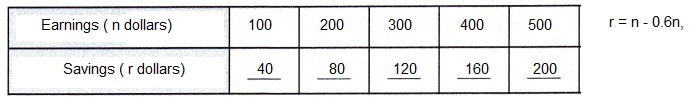Explanation:
Made a table above to show the relationship between r and n. Using values of n = 100, 200, 400, and 500 in my table and substituting in r = n – 0.6n as
if n = 100 then r = 100 – 0.6 X 100 = 100 – 60 = 40,
if n = 200 then r = 200 – 0.6 X 200 = 200 – 120 = 80,
if n = 300 then r = 300 – 0.6 X 300 = 300 – 180 = 120,
if n = 400 then r = 400 – 0.6 X 400 = 400 – 240 = 160 and
if n = 500 then r = 500 – 0.6 X 500 = 500 – 300 = 200.

c) Graph the relationship between n and r in a coordinate plane.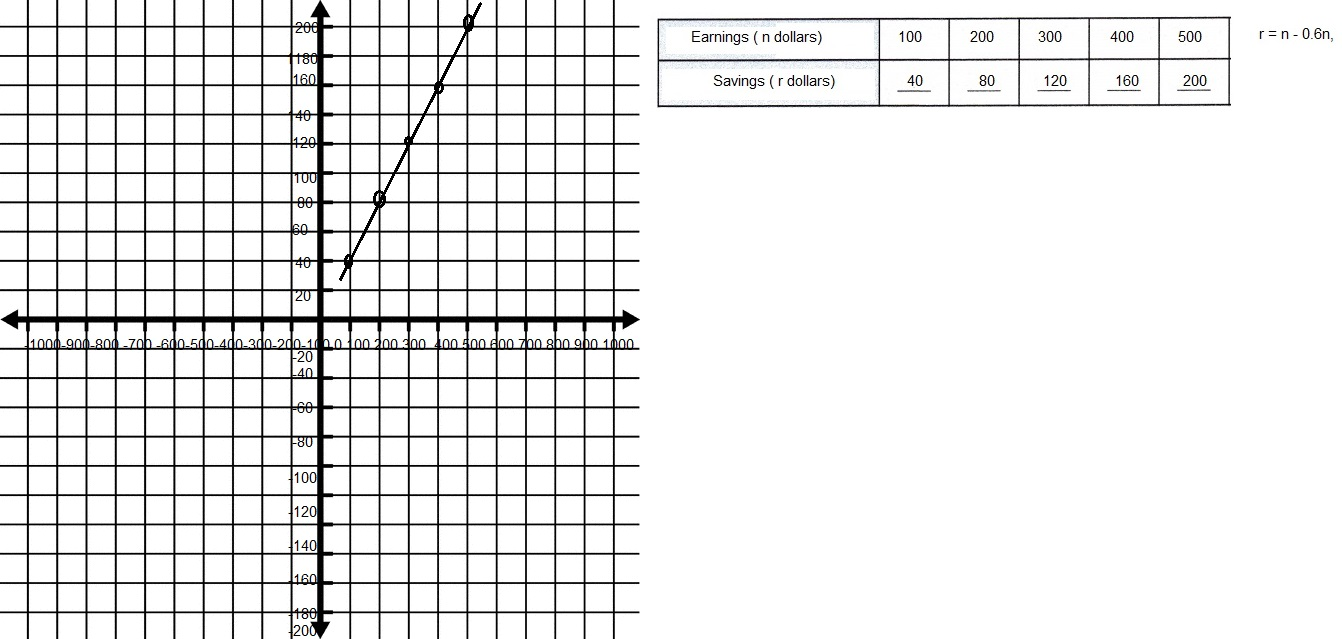Explanation:
Graphed the relationship between n and r in a coordinate plane as  r = n – 0.6n where earnings n dollars on x – axis and savings r dollars on y – axis as shown above.

d) The point (287.5, 115) is on the line you drew in c). Does this point make sense in the situation? Explain.
Yes,

Explanation:
Given the point (287.5, 115) is on the line I drew in c) checking in r = n – 0.6n, if n = 287.5 then r =  287.5 – 0.6 X 287.5 = 287.5 – 172.5 = 115 which matches so this point make sense in the situation.

Question 9.
The side length of a square is t inches. The perimeter of the square is z inches.
a) Express z in terms of t.
z = 4t,

Explanation:
Given the side length of a square is t inches. The perimeter of the square is z inches. Expressing z in terms of t as perimeter of sqaure is 4 X side length so it is z = 4t.

b) Make a table to show the relationship between z and t. Use whole number values of t from 1 to 10.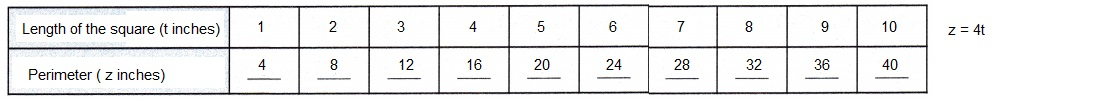Explanation:
Made a table to show the relationship between z and t. Used whole number values of t from 1 to 10 in z – 4t as
if t = 1 then z = 4 X 1 = 4,
if t = 2 then z = 4 X 2 = 8,
if t = 3 then z = 4 X 3 = 12,
if t = 4 then z = 4 X 4 = 16,
if t = 5 then z = 4 X 5 = 20,
if t = 6 then z = 4 X 6 = 24,
if t = 7 then z = 4 X 7 = 28,
if t = 8 then z = 4 X 8 = 32,
if t = 9 then z = 4 X 9 = 36 and
if t = 10 then z = 4 X 10 = 40 shown above.

c) Graph the relationship between z and t in a coordinate plane.